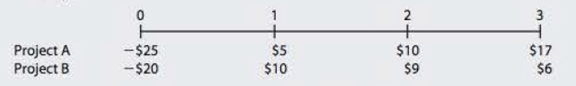Chapter 11, Problem 6PFundamentals of Financial Manageme...

15th Edition
Eugene F. Brigham + 1 other
ISBN: 9781337395250

Solutions

Chapter
SectionFundamentals of Financial Manageme...

15th Edition
Eugene F. Brigham + 1 other
ISBN: 9781337395250
Textbook Problem

NPV Your division is considering two projects with the following cash flows (in millions):a. What are the projects’ NPVs assuming the WACC is 5%? 10%? 15%? b. What are the projects’ IRRs at each of these WACCs? c. If the WACC was 5% and A and B were mutually exclusive, which project would you choose? What if the WACC was 10%? 15%? (Hint: The crossover rate is 7.81%.)

a.

Summary Introduction

To calculate: The NPV of the given projects at 5%, 10 % and 15% WACC.

Introduction:

Net Present Value (NPV): It is a method under capital budgeting which includes the calculation of net present value of the project in which the company is investing. The calculation is done based on the difference between the value of cash inflow and value of cash outflow after considering the discounted rate.

Weighted Average Cost of Capital (WACC): It is the discounted rate that has been calculated by the company on the basis of which present values of cash outflows and inflows are calculated under capital budgeting.

Explanation

Given information:

Project A:

Initial investment for Project A is $25 million. Cash inflow after 1st year is$5 million.

Cash inflow after 2nd year is $10 million. Cash inflow after 3rd year is$17 million.

WACC is 5%, 10% and 15%.

The formula to calculate NPV is:

NPV=InitialInvestment+CashFlow1(1+WACC)1+CashFlow2(1+WACC)2+CashFlow3(1+WACC)3

Substitute $25 for initial investment 5 for CashFlow1 , 10 for CashFlow2 , 17 for CashFlow3 and 5% for WACC in the formula for Project A. NPV=$25+$5(1+5%)1+$10(1+5%)2+$17(1+5%)3=$25+$4.76+$9.07+$14.69=$3.52

The NPV for Project A with 5% WACC is $3.52 million. Substitute$25 for initial investment 5 for CashFlow1 , 10 for CashFlow2 , 17 for CashFlow3 and 10% for WACC in the formula for Project A.

NPV=$25+$5(1+10%)1+$10(1+10%)2+$17(1+10%)3=$25+$4.55+$8.26+$12.77=$0.58 The NPV for Project A with 10% WACC is$0.58 million.

Substitute $25 for initial investment 5 for CashFlow1 , 10 for CashFlow2 , 17 for CashFlow3 and 15% for WACC in the formula for Project A. NPV=$25+$5(1+15%)1+$10(1+15%)2+$17(1+15%)3=$25+\$4

b.

Summary Introduction

To calculate: The IRR of the given projects at 5%. 10% and15% WACC.

Introduction:

Internal Rate of Return (IRR):

It refers to the rate of return that is computed by the company to make a decision of selection of a project for investment. This rate provides the basis for selection of projects with lower cost of capital and rejection of project with higher cost of capital.

c.

Summary Introduction

To explain: Whether the Project A and Project B should be chosen when WACC is 5%, 10% and 15% respectively.

Still sussing out bartleby?

Check out a sample textbook solution.

See a sample solution

The Solution to Your Study Problems

Bartleby provides explanations to thousands of textbook problems written by our experts, many with advanced degrees!

Get Started

Why should policymakers think about incentives?

Brief Principles of Macroeconomics (MindTap Course List)

What is a data flow diagram (DFD)?

Accounting Information Systems

Why is EBIT generally considered independent of financial leverage? Why might EBIT actually be affected by fina...

Fundamentals of Financial Management, Concise Edition (with Thomson ONE - Business School Edition, 1 term (6 months) Printed Access Card) (MindTap Course List)